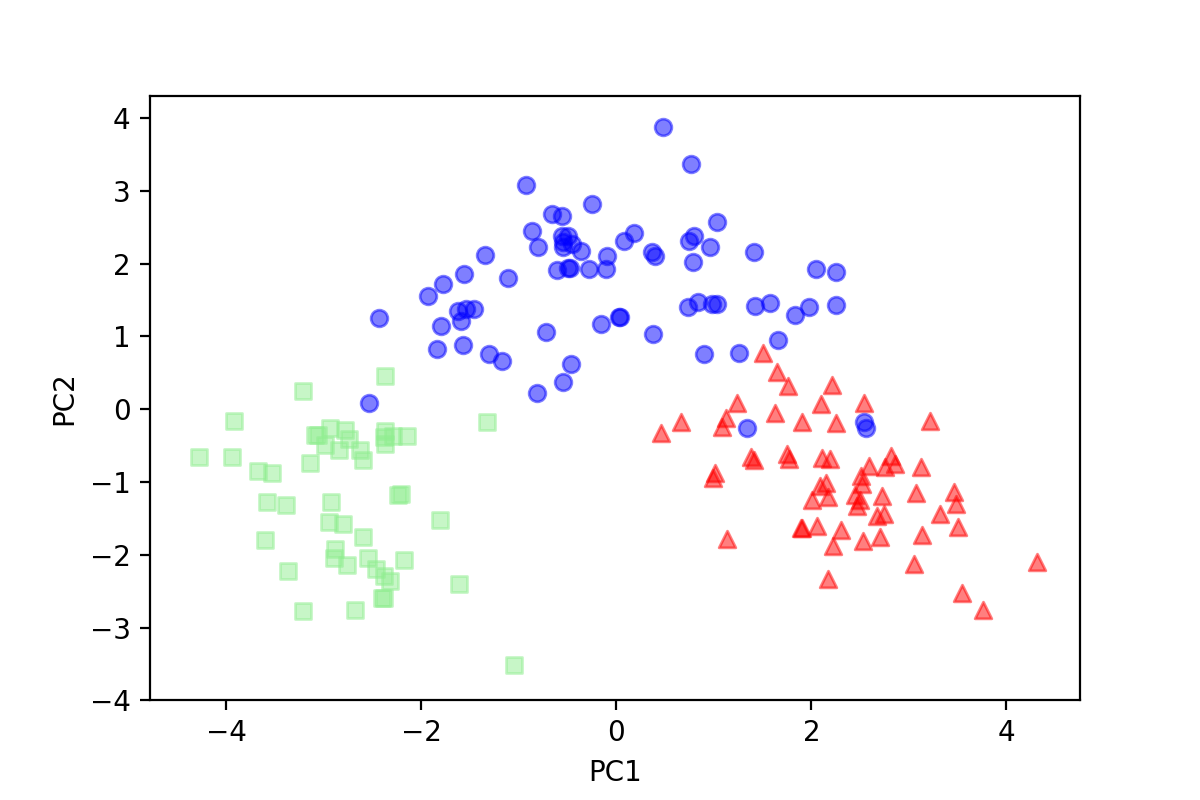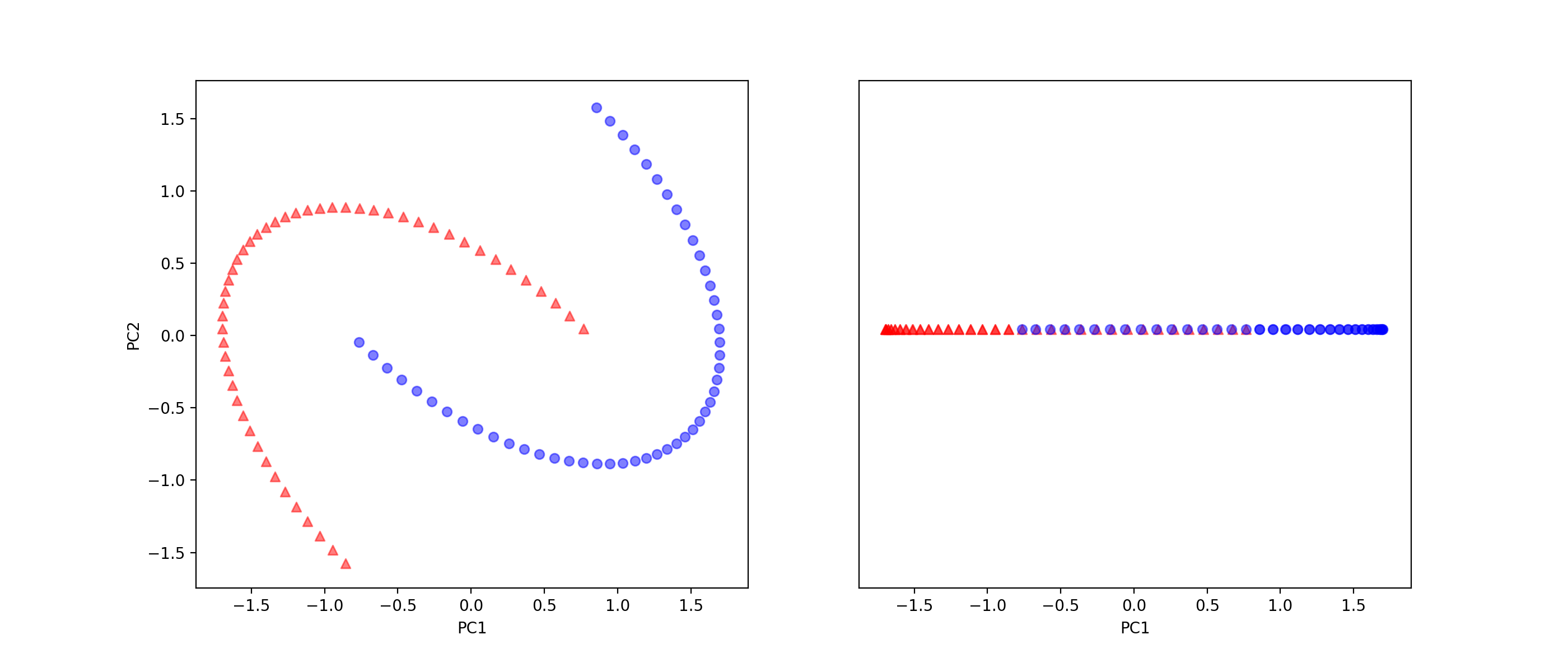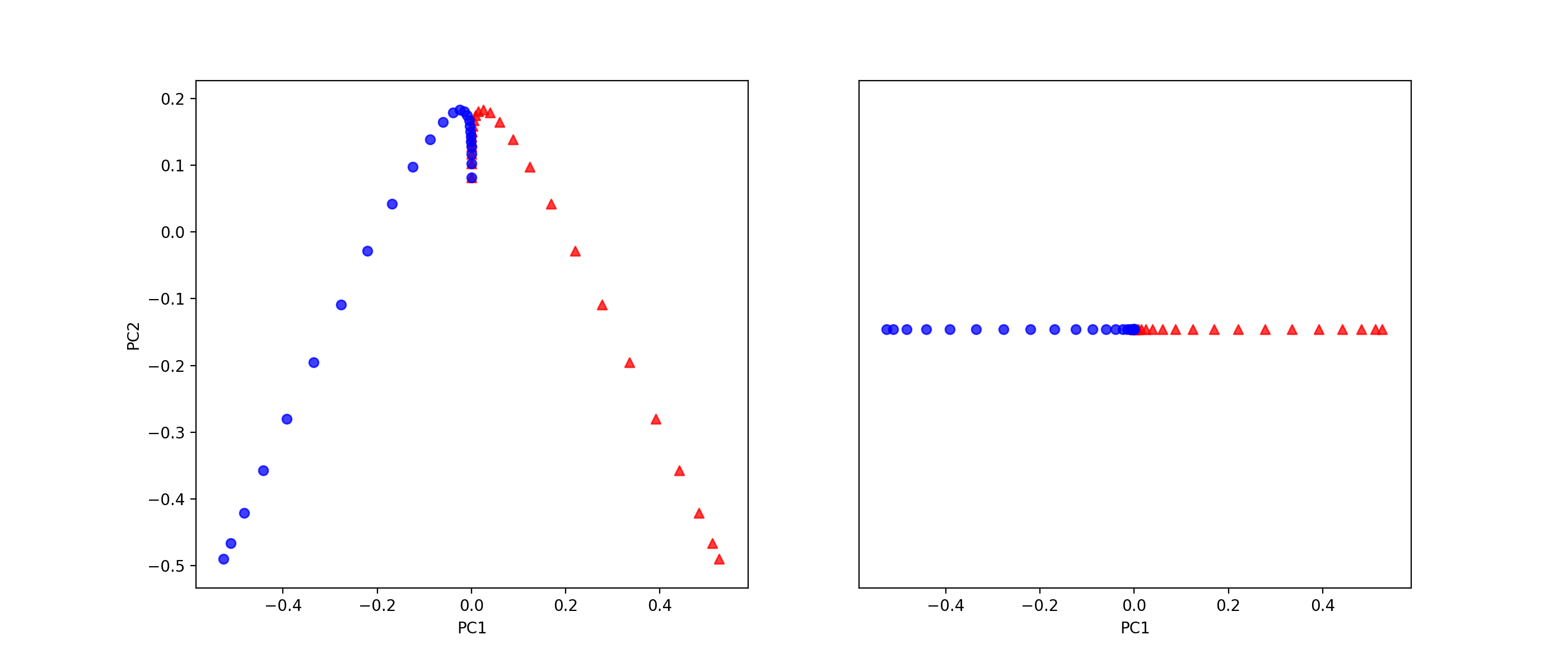PCA的原理

什么是投影xj$x_j$投影到v$v$上的向量为
xj=(||xj||cosθ)v||v||$x_j'=(||x_j||cos\theta)\dfrac{v}{||v||}$

<xj,v>=||xj||||v||cosθ$=||x_j|| \cdot ||v|| \cdot cos\theta$

xj=<xj,v>||v||2v$x_j' = \dfrac{}{||v||^2}v$

xj=<xj,v>v$x_j' = v$

<xj,v>=xTjv=vTxj$=x_j^T \cdot v=v^T \cdot x_j$

投影后的方差

σ2=1N1Ni=1(vTxi0)2=1N1Ni=1(vTxi)(vTxi)$\sigma^2 = \dfrac{1}{N - 1}\sum_{i=1}^{N}(v^Tx_i-0)^2=\dfrac{1}{N - 1}\sum_{i=1}^{N }(v^Tx_i)(v^Tx_i)$

σ2=1N1Ni=1vTxixTiv=vT(1N1Ni=1xixTi)v=vTCv$\sigma^2=\dfrac{1}{N - 1}\sum_{i=1}^{N}v^Tx_ix_i^Tv=v^T(\dfrac{1}{N- 1}\sum_{i=1}^{N}x_ix_i^T)v=v^TCv$

转化为求特征值的问题

maxvTCvs.t.||v||=1$max \quad v^TCv \\ s.t. \quad ||v||=1$

maxvTCvs.t.vTv=1$max \quad v^TCv \\ s.t. \quad v^Tv=1$

f(v,λ)=vTCvλ(vTv1)$f(v,\lambda)=v^TCv-\lambda (v^Tv-1)$

fv=2Cv2λv=0fλ=vTv1=0$\begin{cases}\dfrac{\partial f}{\partial v}=2Cv-2\lambda v=0 \\ \dfrac{\partial f}{\partial \lambda}=v^Tv-1=0 \end{cases}$

{Cv=λv||v||=1$\begin{cases}Cv=\lambda v \\ ||v|| =1\end{cases}$

vTCv=vTλv=λvTv=λ$v^TCv=v^T\lambda v=\lambda v^Tv=\lambda$

符号的表示

C=1N1Ni=1xixTi=1N1[x1,x2,...,xN]xT1xT2...xTN$C=\dfrac{1}{N - 1}\sum_{i=1}^{N}x_ix_i^T=\dfrac{1}{N - 1}[x_1,x_2,...,x_N] \begin{bmatrix}x_1^T \\ x_2^T \\... \\ x_N^T \end{bmatrix}$

xi=x(1)ix(2)i...x(m)i$x_i=\begin{bmatrix}x_i^{(1)} \\ x_i^{(2)} \\... \\x_i^{(m)} \end{bmatrix}$

XT=[x1,x2,...,xN]$X^T=[x_1,x_2,...,x_N]$

C=1N1XTX$C=\dfrac{1}{N - 1}X^TX$

KPCA的原理

C=1N1Ni=1ϕ(xi)ϕ(xi)T=1N[ϕ(x1),...,ϕ(xN)]ϕ(x1)T...ϕ(xN)T$C=\dfrac{1}{N - 1}\sum_{i=1}^{N}\phi (x_i)\phi(x_i)^T=\dfrac{1}{N}[\phi(x_1),...,\phi(x_N)]\begin{bmatrix}\phi(x_1)^T \\ ... \\ \phi(x_N)^T \end{bmatrix}$

XT=[ϕ(x1),...,ϕ(xN)]$X^T=[\phi(x_1),...,\phi(x_N)]$

C=1N1XTX$C=\dfrac{1}{N - 1}X^TX$

K=XXT=ϕ(x1)T...ϕ(xN)T[ϕ(x1),,ϕ(xN)]=κ(x1,x1)κ(xN,x1)...κ(x1,xN)κ(xN,xN)$K=XX^T=\begin{bmatrix} \phi(x_1)^T \\...\\ \phi(x_N)^T \end{bmatrix} [ \phi(x_1) , \cdots ,\phi(x_N)]=\begin{bmatrix} \kappa(x_1,x_1) & ... & \kappa(x_1,x_N) \\ \vdots & \ddots & \vdots \\ \kappa(x_N, x_1) & \cdots & \kappa(x_N,x_N) \end{bmatrix}$

(XXT)u=λu$(XX^T)u=\lambda u$

XT(XXT)u=λXTu$X^T(XX^T)u=\lambda X^Tu$

(XTX)(XTu)=λ(XTu)$(X^TX)(X^Tu)=\lambda (X^Tu)$

v=1||XTu||XTu=1uTXXTuXTu=1uTKuXTu=1uTλuXTu=1λXTu$v=\dfrac{1}{||X^Tu||}X^Tu=\dfrac{1}{\sqrt{u^TXX^Tu}}X^Tu=\dfrac{1}{\sqrt{u^TKu}}X^Tu=\dfrac{1}{\sqrt{u^T\lambda u}}X^Tu=\dfrac{1}{\sqrt{\lambda}}X^Tu$

vTϕ(xj)=(1λXTu)Tϕ(xj)=1λuTXϕ(xj)=1λuTϕ(x1)Tϕ(xN)Tϕ(xj)=1λuTκ(x1,xj)κ(xN,xj)$v^T\phi(x_j)=(\dfrac{1}{\sqrt{\lambda}}X^Tu)^T\phi(x_j)=\dfrac{1}{\sqrt{\lambda}}u^TX\phi(x_j)=\dfrac{1}{\sqrt{\lambda}}u^T\begin{bmatrix} \phi(x_1)^T \\ \vdots \\ \phi(x_N)^T \end{bmatrix} \phi(x_j)=\dfrac{1}{\sqrt{\lambda}}u^T\begin{bmatrix} \kappa(x_1, x_j) \\ \vdots \\ \kappa(x_N, x_j) \end{bmatrix}$

PCA和KPCA在Python中的使用

PCA的使用

import pandas as pd
from sklearn.preprocessing import StandardScaler

x, y = df.iloc[:, 1:].values, df.iloc[:, 0].values
sc = StandardScaler()
x = sc.fit_transform(x)

from sklearn.decomposition import PCA

pca = PCA(n_components=2)
x_pca = pca.fit_transform(x)

import matplotlib.pyplot as plt

plt.scatter(x_pca[y==1, 0], x_pca[y==1, 1], color='red', marker='^', alpha=0.5)
plt.scatter(x_pca[y==2, 0], x_pca[y==2, 1], color='blue', marker='o', alpha=0.5)
plt.scatter(x_pca[y==3, 0], x_pca[y==3, 1], color='lightgreen', marker='s', alpha=0.5)
plt.xlabel('PC1')
plt.ylabel('PC2')
plt.show()KPCA的使用

PCA的使用是有局限性的，如果遇到了，一个像下面这样的线性不可分的数据集，就比较麻烦了。

from sklearn.datasets import make_moons

x2, y2 = make_moons(n_samples=100, random_state=123)

plt.scatter(x2_std[y2==0, 0], x2_std[y2==0, 1], color='red', marker='^', alpha=0.5)
plt.scatter(x2_std[y2==1, 0], x2_std[y2==1, 1], color='blue', marker='o', alpha=0.5)
plt.xlabel('PC1')
plt.ylabel('PC2')
plt.show()x2_std = sc.fit_transform(x2)
x_spca = pca.fit_transform(x2_std)

fig, ax = plt.subplots(nrows=1, ncols=2, figsize=(14,6))
ax.scatter(x_spca[y2==0, 0], x_spca[y2==0, 1], color='red', marker='^', alpha=0.5)
ax.scatter(x_spca[y2==1, 0], x_spca[y2==1, 1], color='blue', marker='o', alpha=0.5)
ax.scatter(x_spca[y2==0, 0], np.zeros((50,1))+0.02, color='red', marker='^', alpha=0.5)
ax.scatter(x_spca[y2==1, 0], np.zeros((50,1))+0.02, color='blue', marker='o', alpha=0.5)
ax.set_xlabel('PC1')
ax.set_ylabel('PC2')
ax.set_ylim([-1, 1])
ax.set_yticks([])
ax.set_xlabel('PC1')
plt.show()from sklearn.decomposition import KernelPCA

kpca = KernelPCA(n_components=2, kernel='rbf', gamma=15)
x_kpca = kpca.fit_transform(x2_std)

fig, ax = plt.subplots(nrows=1, ncols=2, figsize=(14,6))
ax.scatter(x_kpca[y2==0, 0], x_kpca[y2==0, 1], color='red', marker='^', alpha=0.5)
ax.scatter(x_kpca[y2==1, 0], x_kpca[y2==1, 1], color='blue', marker='o', alpha=0.5)
ax.scatter(x_kpca[y2==0, 0], np.zeros((50,1))+0.02, color='red', marker='^', alpha=0.5)
ax.scatter(x_kpca[y2==1, 0], np.zeros((50,1))+0.02, color='blue', marker='o', alpha=0.5)
ax.set_xlabel('PC1')
ax.set_ylabel('PC2')
ax.set_ylim([-1, 1])
ax.set_yticks([])
ax.set_xlabel('PC1')
plt.show()